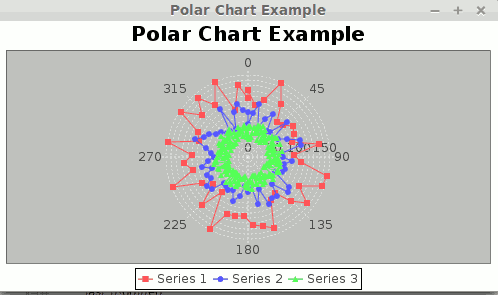# Create Polar Chart Using JFreeChart

Create Polar Chart Using JFreeChart explains about creating a simple polar chart using JFreechart API

A polar chart or polar graph is a circular graph on which data points are displayed using the angle, and the distance from the center point. The X axis is located on the boundaries of the circle and the Y axis connects the center of the circle with the X axis.

Reference -> https://msdn.microsoft.com/en-us/library/dd456623%28v=vs.140%29.aspx

How To Create Polar Chart Using JFreeChart library?

JFreeChart is a free and open source java chart library used for creating professional quality charts. JFreeChart is purely written in java language, we can very easily incorporate JFreeChart in our java standalone and web applications.

Referenece - > http://www.jfree.org/jfreechart/

Here I am showing an example about How to create  a simple Polar Chart using JFreeChart.

Note

1) If you need pie chart example, You can follow Create Pie Chart Using JFreeChart

2) If you need line chart example, You can follow Create Line Chart Using JFreeChart

3) If you need bar chart example, You can follow Create Bar Chart Using JFreeChart

4) If you need polar chart example, You can follow Create Polar Chart Using JFreeChart

5) If you need bubble chart example, You can follow Create Bubble Chart Using JFreeChart

6) If you need wind chart example, You can follow Create Wind Chart Using JFreeChart

###### Required Libraries

Following jar must be in classpath

1. jfreechart-1.5.0.jar
2. jcommon-1.0.24.jar

### pom.xml

```<dependency>
<groupId>org.jfree</groupId>
<artifactId>jfreechart</artifactId>
<version>1.5.0</version>
</dependency>
<dependency>
<groupId>org.jfree</groupId>
<artifactId>jcommon</artifactId>
<version>1.0.24</version>
</dependency>```

We can create following professional quality charts by using jfreechart.

• Single valued Charts such as compass, speedometer, thermometer
• Line Chart
• Pie Chart
• Bar Chart
• Bubble Chart
• Wind Chart
• Polar Chart

## JFreeChart Example (Polar Chart)

import javax.swing.JFrame;

import org.jfree.chart.ChartFactory;
import org.jfree.chart.ChartPanel;
import org.jfree.chart.JFreeChart;
import org.jfree.data.xy.XYSeries;
import org.jfree.data.xy.XYSeriesCollection;

public class JFreeChartPolarChartExample extends JFrame {

private static final long serialVersionUID = 1L;

public JFreeChartPolarChartExample(String applicationTitle) {

super(applicationTitle);

// Creates a sample dataset for polar chart

XYSeriesCollection  dataSet = new XYSeriesCollection();
XYSeries series1 = createRandomData
("Series 1", 77.0, 8.0);
XYSeries series2 = createRandomData
("Series 2", 52.0, 6.0);
XYSeries series3 = createRandomData
("Series 3", 32.0, 2.0);
(series1);
(series2);
(series3);

// Based on the dataset we are creating BubbleChart

JFreeChart polarChart = ChartFactory.createPolarChart("Polar Chart Example", dataSet, true, true, false);

// Adding chart into a chart panel

ChartPanel chartPanel = new ChartPanel(polarChart);

// settind default size

chartPanel.setPreferredSize(new java.awt.Dimension(500, 270));

setContentPane(chartPanel);

}

private static XYSeries createRandomData(final String name, final double baseRadius, final double thetaInc) {

final XYSeries series = new XYSeries(name);

for (double coeff = 0.0; coeff < 365.0; coeff += thetaInc) {

}

return series;

}

public static void main(String[] args) {

JFreeChartPolarChartExample chart = new JFreeChartPolarChartExample("Polar Chart Example");
chart.pack
();
chart.setVisible
(true);

}
}
###### Output